# Quantized Energy States

The electrons in free atoms can only be found in certain discrete energy states. These sharp energy states are associated with the orbits or shells of electrons in an atom, e.g., a hydrogen atom. One of the implications of these quantized energy states is that only certain photon energies are allowed when electrons jump down from higher levels to lower levels, producing the hydrogen spectrum. The Bohr model successfully predicted the energies for the hydrogen atom, but had significant failures that were corrected by solving the Schrodinger equation for the hydrogen atom.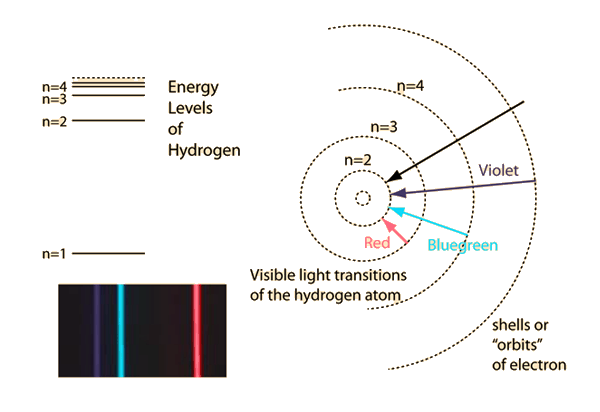### Scaled energy levels

Index

Bohr model concepts

 HyperPhysics***** Quantum Physics R Nave
Go Back

# Angular Momentum Quantization

In the Bohr model, the wavelength associated with the electron is given by the DeBroglie relationship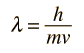and the standing wave condition that circumference = whole number of wavelengths. In the hydrogenic case, the number n is the principal quantum number.These can be combined to get an expression for the angular momentum of the electron in orbit. (Note that this assumes a circular orbit, a generally unwarranted assumption.)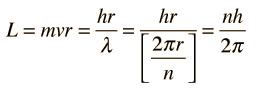Use in Bohr orbit

Thus L is not only conserved, but constrained to discrete values by the quantum number n. This quantization of angular momentum is a crucial result and can be used in determining the Bohr orbit radii and Bohr energies.
Index

Bohr model concepts

 HyperPhysics***** Quantum Physics R Nave
Go Back

# Classical Electron Orbit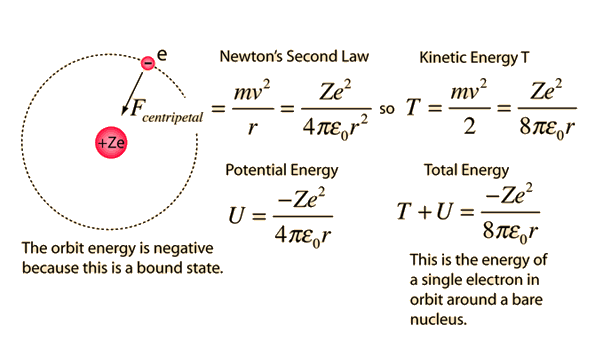In the Bohr theory, this classical result was combined with the quantization of angular momentum to get an expression for quantized energy levels.

### Electric potential

Index

Bohr model concepts

 HyperPhysics***** Quantum Physics R Nave
Go Back

# Bohr Orbit

Combining the energy of the classical electron orbit with the quantization of angular momentum, the Bohr approach yields expressions for the electron orbit radii and energies: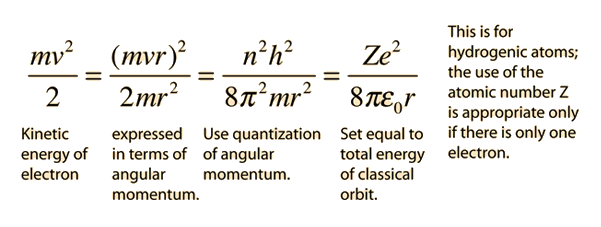Substitution for r gives the Bohr energies and radii: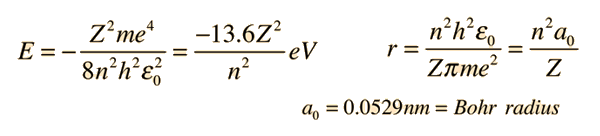Although the Bohr model of the atom was shown to have many failures, the expression for the hydrogen electron energies is amazingly accurate. The Schrodinger equation for the hydrogen atom actually gave the same energies, so the Bohr model was a helpful step along the way to developing a quantum mechanical model for hydrogen.

### Associated electron wavelengths

Index

Bohr model concepts

 HyperPhysics***** Quantum Physics R Nave
Go Back

# Wavelengths for Different States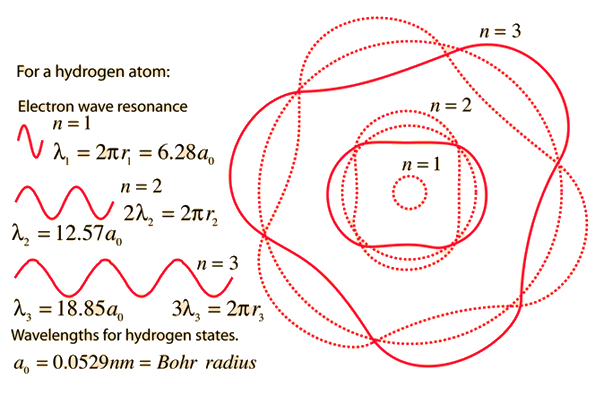### Bohr model of the atom

Index

Bohr model concepts

 HyperPhysics***** Quantum Physics R Nave
Go Back

# Failures of the Bohr Model

While the Bohr model was a major step toward understanding the quantum theory of the atom, it is not in fact a correct description of the nature of electron orbits. Some of the shortcomings of the model are:

1. It fails to provide any understanding of why certain spectral lines are brighter than others. There is no mechanism for the calculation of transition probabilities.

2. The Bohr model treats the electron as if it were a miniature planet, with definite radius and momentum. This is in direct violation of the uncertainty principle which dictates that position and momentum cannot be simultaneously determined.

The Bohr model gives us a basic conceptual model of electron orbits and energies. The precise details of spectra and charge distribution must be left to quantum mechanical calculations, as with the Schrodinger equation.

### Bohr model of the atom

Index

Bohr model concepts

 HyperPhysics***** Quantum Physics R Nave
Go Back